# Statics and dynamics of malaria infection in Anopheles mosquitoes

## Abstract

The classic formulae in malaria epidemiology are reviewed that relate entomological parameters to malaria transmission, including mosquito survivorship and age-at-infection, the stability index (S), the human blood index (HBI), proportion of infected mosquitoes, the sporozoite rate, the entomological inoculation rate (EIR), vectorial capacity (C) and the basic reproductive number (R0). The synthesis emphasizes the relationships among classic formulae and reformulates a simple dynamic model for the proportion of infected humans. The classic formulae are related to formulae from cyclical feeding models, and some inconsistencies are noted. The classic formulae are used to to illustrate how malaria control reduces malaria transmission and show that increased mosquito mortality has an effect even larger than was proposed by Macdonald in the 1950's.

## Review

Mathematical models have played an important role in understanding the epidemiology of malaria and other infectious diseases . Models provide concise quantitative descriptions of complicated, non-linear processes, and a method for relating the process of infection in individuals to the incidence of infection or disease in a population over time. Important insights have come from dynamic and static analysis of these models [4, 5]. Dynamic analysis focuses on changes in the incidence or prevalence of an infectious disease in a population over time. Statics involve populations at a steady state. Dynamic epidemic processes leave a signature in a population that can be examined statically, often from static analyses of age-specific patterns in epidemiological status, based on an appropriate cross-section of the population at a point in time – a snapshot of a population, stratified by age provides information about its historical dynamics [4, 5]. The assumption that a population is at dynamic equilibrium and has a stationary age distribution is frequently violated in practice, but it provides a useful starting point for more sophisticated analysis. Combining mathematical modelling with statistical analysis allows the use of static patterns to understand dynamics; basic epidemiological parameters can be estimated in the absence of many years of time series data. The relationship between the statics and dynamics of Plasmodium infections in female Anopheles mosquitoes has been a focal point in classic studies of malaria transmission . For mosquitoes, it is easier to measure parity, the reproductive age of the adult mosquito, than the chronological age of the mosquito, the time since adult emergence [6, 7]. Saul et al. reformulated classic models for static age-infection relationships as cyclical feeding models; i.e. the models were formulated in terms of parity. They used the models to analyse mosquito statics, derive a formulae for the sporozoite rate, and define individual vectorial capacity . Killeen et al. followed a similar line of thinking and estimated the entomological inoculation rate (EIR) from studies of mosquito populations . Both models use the gonotrophic cycle of the mosquito to mark time, but the underlying assumptions about mosquito populations are essentially identical to those of Ross and Macdonald [1, 2]. The parallel approaches have led to a proliferation of formulae.

In this paper, the classic formulae in malaria are reviewed and re-derived including explicit and simple formulae for the statics of Plasmodium infection in mosquitoes such as mosquito survivorship, the human blood index (HBI), the stability index (S), the proportion of mosquitoes that are infected (i.e. a mosquito with detectable Plasmodium), and the proportion of mosquitoes that are infectious (i.e. the sporozoite rate). These quantities are related to transmission through EIR, vectorial capacity, and the basic reproductive number, R0. Next, simple dynamic models are formulated that are slightly different from the classic formulations; these modified equations are consistent with the statics of infection. The focus is on the role of mosquitoes in malaria transmission; for human infections, the equations use simple assumption of Ross, ignoring super-infection and immunity [1, 2, 10]. Super-infection and immunity have been reviewed in greater depth by others [11, 12]. Seven independent quantities must be estimated for a simple malaria transmission model. Of these, five can be estimated from mosquito statics. Finally, the classic malaria models are related directly to cyclical feeding models.

### Statics of mosquito infections

As in the models used to derive the classic formulae in malaria epidemiology, the following derivations assume that mosquito populations are homogeneous, ignore mosquito senescence, and assume that adult mosquito population size is constant. Moreover, the formulae assume that mosquitoes bite humans at random and uniformly. Also, the formulae assume that an infected mosquito never becomes uninfected, and so focus on the initial infection. These assumptions, which are called the classic assumptions, are useful approximations or idealizations that form a starting point for more complicated analysis. The classic assumptions are frequently violated in real mosquito populations. For example, mosquito density fluctuates seasonally; emergence and survivorship may depend on environmental factors, such as rainfall, temperature and humidity. Adult mosquitoes may senesce, or there may be intrinsic differences in mortality rates, called demographic frailty. Mosquitoes bite some people more than others , either due to inherent mosquito preferences  or proximity to larval habitat .

#### Survivorship & Lifespan

The classic models make simple assumptions about mosquito survivorship, a term from demography that is defined as the proportion of individuals from a cohort that surviving to a given age, and typically presented as a survivorship curve. Let g denote the "force of mortality," the per-capita daily death rate of a mosquito. The assumption that survivorship is constant over the mosquito lifespan implies an exponential distribution of survival times (i.e. a hazard model). The proportion of a cohort of mosquitoes that survives to age A is

λ(A) = e-gA.     (1)

Thus, the probability that an individual mosquito survives one day is p = e-g, or equivalently g = - ln p. The median survival time (i.e. population half-life) is ln 2/g. In general, the proportion of mosquitoes that dies at age A is (A), and the average lifespan of a mosquito is: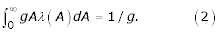Put another way, if g is the force of mortality, then 1/g is the average mosquito lifespan, or the expected waiting time to death. Mosquito lifespan is one component of the lifetime transmission potential of an individual mosquito; human feeding rate is the other.

#### Human Feeding, Stability Index & HBI

How many bites on a human will a mosquito take during its lifetime? Let f denote the mosquito feeding rate; the interval between blood meals is assumed to be equivalent to the time interval between successive ovipositions, denoted 1/f. Further, let Q denote the proportion of bites that are taken on humans, a parameter that can be estimated from the proportion of mosquitoes that have ever fed on a human, called the human blood index, HBI. The human feeding rate, a is the expected number of bites on humans per mosquito, per day; a = Qf. Since a mosquito lives 1/g days and bites a human once every a days, a mosquito bites humans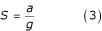times over the course of its lifetime; S is called the "stability index" [2, 19]. By assumption, the life expectancy of a mosquito that has already lived 10 days is exactly the same as a recently emerged mosquito, 1/g days. Thus, S is also the number of bites given by a mosquito after it has become infectious. The expected number would be lower in a population with senescence and higher in a population with demographic frailty.

What fraction of mosquitoes of age A have bitten a human? A mosquito of age A is expected to have given f A bites, of which a A = fQA are on humans. Following a cohort of recently emerged mosquitoes over time, the proportion of a surviving mosquitoes of age A that has ever bitten a human is:

η(A) = 1-e-aA.     (4)

The proportion of mosquitoes in a population that has survived to age A and bitten a human is η (A)λ(A), and HBI is: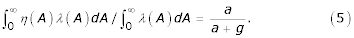Note that the proportion of fed mosquitoes f/(f + g), can be derived in a similar way, assuming a = f. Thus, the HBI is a simple function of the mosquito lifespan and the human feeding rate. It can be understood as a ratio of two waiting times: the waiting time to either the first human bite or death 1/(a + g) and the waiting time to the first human bite among surviving mosquitoes (1/a).

#### Proportion of Infected Mosquitoes

What is the probability that a mosquito becomes infected over the course of its lifetime? Infected mosquitoes, in this case, are those with any of the parasite developmental stages, e.g. oocysts or sporozoites. Mosquitoes become infected upon biting an infectious human. Let X denote the proportion of humans who are infectious, and since this is a static analysis, X is assumed to remain constant. Let c denote the transmission efficiency from an infectious human to an uninfected mosquito; in other words, c is the probability that an uninfected mosquito becomes infected after biting an infectious human. Thus, mosquitoes become infected at the rate acX. Following a cohort of recently emerged mosquitoes over time, the proportion of surviving mosquitoes of age A that have ever become infected is:

v(A) = 1 - e-acXA.     (6)

The proportion of the original mosquito cohort that is alive and infected at age A is v(A)λ(A), and the proportion of infected mosquitoes, Y, is: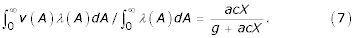Thus the proportion of infected mosquitoes is a ratio of two waiting times: the waiting time to either death or infection, 1/(g + acX), and the waiting time to infection among surviving mosquitoes 1/(acX).

#### Proportion of Infectious Mosquitoes

What is the probability that a mosquito survives to become infectious? Infectious is defined as a mosquito has sporozoites in its salivary glands. Let n denote the length of the incubation period, from ingestion of gametocytes to becoming infectious, called the entomological incubation period. That is, only mosquitoes that have survived at least n days could be infectious; the probability of surviving n days is P e = e-gn.

What is the probability that a mosquito becomes infectious over the course of its lifetime? The proportion of mosquitoes of age A that are infectious is: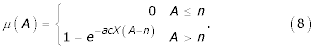The proportion of a mosquito cohort that is alive and infectious at age A is μ (A)λ(A). Thus, the proportion of mosquitoes that are infectious, Z, (also called the "sporozoite rate"), or, equivalently, the probability that an individual mosquito ever becomes infectious, is: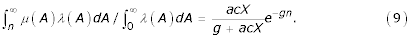This expression is similar to the one given by Macdonald; they are identical if c = 1 (see page v, formula (7) in ). Note that it is simply the product of the probabilities of ever becoming infected and, then, surviving the incubation period, Z = YP e .

The lifetime transmission potential of a mosquito is defined as the expected number of new infections that would be generated by a newly emerged adult. Let b denote the transmission efficiency from an infectious mosquito to an uninfected, susceptible human; in other words, b is the probability that an uninfected human becomes infected after being bitten by an infectious mosquito. The expected reproductive output of a cohort of mosquitoes at age A is baμ(A)λ(A). Lifetime transmission potential, denoted β, is integrated over a mosquito lifetime: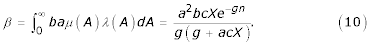Thus, lifetime transmission potential is the product of the probability that a mosquito becomes infected, Y, the probability that an infected mosquito survives to become infectious, P e , transmission efficiency, b, and the stability index, S.

Lifetime transmission potential for a mosquito is a function of the proportion of a human population that is infectious, X, as well as the other parameters. The curve β (X,...) is concave down, with initial slope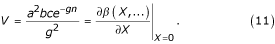Thus, VXβ (X); the two differ by the factor (1 + acX/g)-1 [= g/(g + acX)]. Note that it is the product of three quantities: the square of the stability index, S2, the net transmission efficiency bc, and the probability of surviving the incubation period P e . Stability index is squared because transmission requires at least two bites, one for infection and one to transmit.

The formula V describes the total contribution to vectorial capacity of a single mosquito over its lifetime, and it is used to estimate the critical mosquito density (see below). Note that Saul, et al.  define a closely related formula called individual vectorial capacity (IC):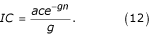IC is defined as the expected number of infectious bites from a single vector after feeding on an infectious host. It is the product of three quantities: the probability that a vector becomes infected by that bite (probability c), the probability that it survives the incubation period, P e , and the stability index, S. Thus, V counts infectious bites that would result in an infection, while IC counts only infectious bites; V begins counting when an adult mosquito emerges, while IC begins counting after a mosquito has bitten an infectious human.

#### EIR & Vectorial Capacity

EIR depends on the statics of a mosquito population as well as the density of mosquitoes. By definition, EIR is the number of infectious bites received per day by a human, or equivalently, the human biting rate multiplied by the sporozoite rate. If mosquitoes emerge at the constant rate ε per human per day, then the equilibrium mosquito density per human is m = ε/g.

EIR can be written in several equivalent ways. First, EIR = maZ = εSZ; Z is the sporozoite rate, the proportion of infectious mosquitoes, and ma = εS is called the human biting rate (HBR), the number of bites per human per day, the product of the the density of mosquitoes per human, and the human feeding rate. (Note that "human feeding rate" counts the number of bites on a human, per mosquitoes, per day, while "human biting rate" counts bites on humans, per human, per day.) Next, EIR can be rewritten in terms of the lifetime transmission potential of a mosquito – a population of humans would receive the same number of infectious bites if each emerging mosquito delivered all of its infectious bites upon emergence and promptly died ; thus EIR = εβ = mgβ. Finally, EIR can be rewritten in terms of the basic parameters. Thus,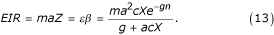(Again Macdonald gives a nearly identical expression, setting c = 1: see page v, formula (9) in ). Note that any one of these formulae for EIR is correct and could be generated from an understanding of the underlying entomological parameters.

Note that EIR, like the sporozoite rate, is a function of the proportion of humans who are infectious. In contrast, vectorial capacity, C, describes the transmission potential of a mosquito population in the absence of Plasmodium . Vectorial capacity is the expected number of humans infected per infected human, per day, assuming perfect transmission efficiency (i.e. b = c = 1):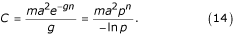Many authors use an alternative definition for vectorial capacity, cC, the number of infectious bites that originate from all the bites on a single infected human in a day . Note that vectorial capacity is not a function of the sporozoite rate, or of the proportion of humans who are infectious, X. Thus, vectorial capacity is independent of X while EIR is dependent on X. In other words EIR does not provide an independent estimate of the proportion of humans who are infected (the "parasite rate") because its calculation requires information about the proportion of humans who are infectious. EIR gives an estimate at a single point in time of the waiting time to the next infectious bite. Note that EIR and vectorial capacity are closely related, since EIR can be regarded as a function of X, and: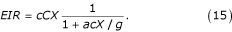Moreover,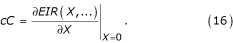That is, vectorial capacity (multiplied by the transmission efficiency c) gives the slope of the relationship between EIR and the prevalence of infection in humans when malaria is virtually absent: cCXEIR when X is small; the approximation cCX is always larger than EIR by the factor (1 + acX/g)-1. Finally, note that the "force of infection" is bEIR; some infectious bites fail to produce an infection. Thus, EIR is only proportional to the rate that new infections occur. Thus, bEIR is the inverse of the expected waiting time to infection for uninfected individuals, or in a population not at steady state, the best estimate at a point in time.

Note that vectorial capacity can be rewritten as the product of the scaled mosquito emergence rate, the stability index squared, and the probability of surviving the infectious period:

C = εS2P e .     (17)

Note the parallels between formulae summarizing entomological aspects of transmission. For example, εV = bcC and εβ = bEIR. The formulae V and β describe transmission rates per mosquito over a lifetime, while cC and EIR describe the number of infectious bites per human. Also, VXβ and bcCXbEIR. Thus, V and bcC measure transmission when X ≈ 0 while β and EIR describe transmission (statically) as a function of X.

### Dynamics & control

Having described the statics of mosquito populations and defined the parameters (see Table 1) an equation for the population dynamics of malaria infection in mosquito populations is the following: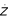= acX (e-gn- Z) - gZ.     (18)

This equation ignores the time lag introduced by incubation (parasite development from ingested gametocytes to salivary-gland sporozoites) but incorporates mosquito mortality during this period. Uninfected mosquitoes become infected at the rate acX, but only the portion that would survive the infectious period, e-gn, become infectious. This model differs slightly from others (see Appendix 1). The equation gives a correct formulation for R0, and the proportion of infectious mosquitoes is correct; at equilibrium (setting= 0, and solving for Z) Eq. 8 is recaptured.

The dynamical model is completed using the classic assumptions about the epidemiology of malaria in humans. Let r denote the recovery rate for humans; this is equivalent to assuming that humans remain infectious for 1/r days after initially becoming infectious, regardless of super-infection and the effects of acquired immunity [1012, 22]. The change in the proportion of infectious humans is described by: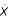= mabZ(1-X)-rX     (19)

This equation ignores the time lag necessary for parasite development from inoculated sporozoites to circulating gametocytes, and human mortality that would occur during this period.

Mosquitoes play a critical role in the life cycle of Plasmodium, but mosquito populations have a relatively rapid turnover. For example, the incubation period in mosquitoes is ignored (1–2 weeks); so is the longer delay in humans (2–3 weeks). Mosquitoes complete a generation during the delay in humans, and, as a consequence, the proportion of mosquitoes that are infectious changes rapidly in response to changes in the proportion of humans that are infectious. Thus, ignoring the time lags, the expression for EIR can be substituted into Eq. 13, to derive a single equation for the dynamics of the proportion of humans that are infectious: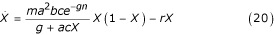This equation de-emphasizes mosquitoes, per se, by reducing their role to a single function describing transmission. A simpler equation can be derived using the approximation: bEIRbcCX:= bcCX(l-X)-rX     (21)

A similar equation, omitting b, was discussed by Dye and Williams . The equation gives a biased estimate for the proportion of humans at equilibrium who are infectious, since bcCX overestimates bEIR by the factor (1 + acX/g)-1. The bias introduced by using vectorial capacity must be weighed against the added mathematical simplicity of the model. In line with the premise that "all models are wrong, but some are useful,"  the choice of equation 20, 21, or some other model should depend on how the model is to be used.

In short, the role of mosquitoes in transmitting human malaria can be reduced to a simple functional relationship describing transmission intensity, with parameters that can be estimated from studying the statics of mosquito populations.

#### Threshold Criteria

The basic reproductive number, R0, provides a threshold criterion. Conceptually, R0 was defined as the expected number of infected humans per infected human, or the number of infected mosquitoes per infected mosquito. The basic reproductive number, R0 is derived from the coupled

Eqs. (18,19):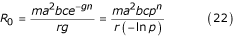(using the "next generation" matrix, this expression is called (R0)2, see [24, 25].) Note that R0 computed from either Eq. 20 or Eq. 21 is identical to 22. This formula differs from more traditional expressions for R0 by including the transmission-efficiency parameter c. For example, Macdonald derives what is otherwise the same expression (his expression for z0 is identical if c = 1: page viii, formula (21) in ); his formula is widely used (e.g. see ).

The threshold can be expressed in various ways that are entomologically and epidemiologically meaningful. Since the basic number is relevant exactly when malaria is absent (i.e. X ≈ 0) thresholds can also be expressed in terms of the basic reproductive number, vectorial capacity, or the transmission potential of an individual mosquito:

R0 = bcC/r = εV/r = mgV/r > 1.     (23)

Rearranging, the threshold can be rewritten as bcC > r; malaria can invade if people can become infected faster than they can recover. How many mosquitoes must be present to sustain an epidemic? The answer is given in terms of scaled emergence, ε >r/V or scaled mosquito density, m >r/(gV). Macdonald called this the critical density of mosquitoes ().

#### Proportion of Infected Humans

The equations can be used to derive the static relationships for the proportion of infected humans. Using equation 20, the prevalence of infection in humans at equilibrium, denoted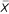, can be written as a function of EIR, C, or R0: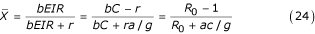The shape of these curves is illustrated in Figure 2. Note that each of these curves is concave down (i.e. the second derivative is negative, and the curve would shed water like an umbrella not hold it like a cup) and asymptotes at= 1 for high EIR (or C or R0). The relationship between vectorial capacity or the basic reproductive number is more complicated:= 0 unless bC >r or R0 > 1. When the force of infection exactly balances the rate of recovery (bEIR = r), exactly half of the human population is infected. In other contexts, this point is called a half-saturation constant, and it determines the shape of the curve.

#### Malaria Control

The classic formulae provide quantitative insights into the entomological aspects of malaria transmission relevant to malaria control. The most critical insights come directly from the formula for R0, that is rewritten using scaled mosquito emergence: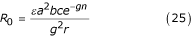Reductions in mosquito emergence, from ε to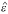, or in the duration of the human infectious period, from 1/r to 1/, have linearly proportional effects on transmission. That is, if the change is expressed relative to the baseline, θ = ε/or φ =/r, R0 is reduced by the factor θ or φ (e.g θ = 2 would imply that mosquito emergence had been cut in half). Reductions in the human feeding rate from a tohave quadratic effects: if ψ = a/, R0 is reduced by the factor ψ2.

Increases in mosquito mortality from a baseline value of g to a post-control value ofhave three effects. First, mosquito density is reduced to from ε/g to ε/, a factor of g/(Macdonald ignored this effect ). Second, the probability of surviving the incubation period is reduced from e-gnto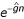, a factor of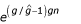. Third, the number of bites per infectious mosquito is reduced from a/g to a/, an additional factor of g/. Thus, if mortality is increased from the baseline value by a factor ζ = g/, R0 is reduced by the total factor egn(ζ-1)/ζ2. The longer the incubation period, the larger the effect. If the incubation period is roughly equal to the average mosquito lifespan (i.e. gn ≈ 1), and adult mortality is doubled, the reduction in R0 is 22e, a factor of about 11; tripling adult mortality would reduce R0 by a factor 32e2 ≈ 67! Single control measures often affect more than one aspect of malaria transmission, as does integrated malaria control. The important point, long understood but often forgotten, is that the benefits are multiplicative. For example, a net reduction of 50% in mosquito emergence (θ = 2) combined with a doubling in adult mortality (ζ ≈ 2, again assuming gn ≈ 1) reduces R0 by a factor of θζ2e ≈ 22. A more ambitious programme that doubled adult mortality, ζ ≈ 2, reduced emergence by 90%, θ ≈ 10, delayed biting by 25%, ψ = 4/3, and reduced the human infectious period by 90% through mass drug administration, φ = 10, would reduce R0 by a factor of φθψ2ζ2e ≈ 1, 932. If such results could be achieved globally, malaria would be eradicated.

Vectorial capacity is far removed from the costs and benefits of actual control programmes, but the analysis suggests that mortality of adult mosquitoes is particularly sensitive to control. Also, since the benefits are multiplicative, integrated malaria control may be the most effective means of control over the long-run.

### Parameter estimation

Malaria surveillance requires seven parameters for the simplest models of malaria transmission (see Table 1). Assuming that human population density is well-characterized, it is necessary to know the density of mosquitoes, the human feeding rate (a), the average mosquito lifespan (1/g), the length of the incubation period (n), the transmission efficiencies (b and c), and the average human infectious period (1/r). Many of these parameters are difficult to estimate directly, but indirect parameter estimates can often be calculated from estimates of independent quantities that are measured directly. For example, mosquito lifespan in a constant population can be estimated in several ways [6, 19, 27]. Once mosquito lifespan has been estimated, human feeding rate can be calculated from an estimate of the human blood index (Eq. 5). Once g and a are known, cX can be estimated from the proportion of mosquitoes that are infected (Eq. 7). Next, the incubation period (n) can be calculated from an estimate of the sporozoite rate (Eq. 8; also see page xvi, formula 69 in ). Transmission efficiencies are difficult to estimate independently, but an estimate of the proportion of humans with circulating gametocytes, X, would provide an independent estimate of c. Some of these parameters may be relatively constant for vector species; were this true, those values would need to be estimated just once, reducing the effective number of parameters to be estimated thereafter.

Other shortcuts may exist. Depending on the purpose of a study, for instance, it may be sufficient to estimate cX without knowing c . The "gold standard" method for estimating EIR involves estimating two quantities directly, the human landing rate (approximately equal to the human biting rate, ma) and the sporozoite rate (Z), but in this case the extrapolation of such estimates to other places or times may be of limited utility.

An alternative is detailed study of mosquito populations. In practice, it is more difficult to measure the chronological age of a mosquito than its parity, the number of ovipositions it has completed [6, 7]. An explicit method for relating transmission parameters to mosquito statics in cyclical feeding models is given by Saul et al. . Thus, cyclical feeding models have been formulated in terms of the reproductive age of the mosquito [8, 9].

Parameter values from cyclical feeding models have a one-to-one mapping onto parameters for models formulated in continuous time, assuming a mosquito of chronological age A has reproductive age τ = A/f. In particular, P f = e-g/fis the probability a mosquito survives exactly one feeding cycle. Thus, cyclical feeding models and the classic models can be compared directly by rescaling time and plotting λ(τ) and μ(τ) for τ = 0,1, 2, 3,... (see Figure 1 and Appendix 2). The chronological age of a mosquito with parity τ depends on time required to find hosts and successfully feed as well as the time required to find oviposition sites and successfully oviposit. Thus, cyclical feeding models and the classic models are both approximations of a more complicated reality.

## Conclusions

Classic formulae for the entomological inoculation rate, basic reproductive number R0, vectorial capacity, and prevalence of infection in humans (the proportion of humans who are infected, or parasite rate) are usually based on the same simplifying assumptions, whether they are formulated as cyclical feeding models or as dynamical equations. The classic assumptions and corresponding models provide a starting point for quantifying malaria transmission and for relating static and dynamic aspects of malaria infection in humans and mosquitoes. They should be regarded as idealizations, serving a purpose similar to that of the Hardy-Weinberg equations in population genetics. The underlying epidemiology of malaria is, of course, more complicated.

The logic of modelling is a priori, from putative cause to effect; the formulae would be correct provided the underlying assumptions were reasonably good approximations to reality and the parameters could be estimated without introducing too much bias . Discrepancies in the actual relationship between EIR (or R0 or vectorial capacity) and the prevalence of infection in humans could be due to sampling error, measurement error, variability in mosquito populations, human immunity, super-infection, heterogeneity in human or mosquito populations, or something else. Discrepancies are not reasons to reject mathematical models; if it is important to have some quantitative understanding of malaria transmission, mathematical models are necessary. Instead, discrepancies between model predictions and reality point out shortcomings in the theory; some of the assumptions are unrealistic for a particular population at a particular time, but which ones? Model building and model selection integrated with field studies will produce models with the appropriate level of complexity.

Which important factors have been omitted from malaria models that must be included? The models of Ross and Macdonald have not performed well in places where malaria is endemic . Ross-Macdonald models may perform well for analyzing thresholds, but these models assume that human biting is evenly distributed. In reality, mosquitoes bite some humans more than others because they are more attractive , or live close to larval habitat [17, 18] which has a surprisingly large impact on the basic reproductive number [13, 29]. Mosquito survivorship, adult emergence rate, feeding rate, and parasite sporogony may vary with temperature, humidity and rainfall, generating wide fluctuations in the density of mosquitoes, proportion of infectious mosquitoes, and EIR. Mosquito populations may senesce or be subject to demographic frailty. The problems of estimating survivorship in a changing population with frailty, senescence, and migration may be intractable. These problems with parameter estimation are still more complicated when several vector species contribute to malaria transmission in an area.

Understanding malaria control through R0 or vectorial capacity provides important qualitative insights into quantitative phenomena. The analysis suggests that the benefits of killing adult mosquitoes are disproportionately high (even higher than suggested by Macdonald ). Integrated control and control measures with multiple effects, such as insecticide-impregnated bed-nets that delay biting and increase adult mortality, may be particularly effective since the benefits are multiplicative. On the other hand, there may be practical limits on increasing mosquito mortality, and benefits may not increase linearly with expenditures. In other words, while the enormous potential benefits of increased adult mortality argue that insecticide-impregnated bed-nets and residual indoor spraying should always be considered, there may be constraints. Indeed, the global DDT-based campaign failed to eradicate malaria due, in part, to the evolution of insecticide resistance and avoidance. Since adult mosquitoes fly long distances but larval habitat is fixed, larval control might be more effective in relative terms; the relative benefits would vary with the larval ecology of the vectors . Furthermore, optimal allocation in integrated malaria control may require a strategy that changes allocations over time. Environmental engineering approaches to larval control may require high initial investments but relatively low-cost maintenance, for example; long-term reductions in adult emergence multiply any subsequent benefits of other measures.

The classic formulae for the stability index, R0, C, and EIR were instrumental in shaping and guiding the DDT-based campaigns to eradicate malaria. Although global eradication failed, in many places the programmes succeeded in substantially reducing mortality and morbidity by reducing transmission. Current malaria control programmes do not explicitly aim to eradicate malaria or even reduce transmission, per se, but these formulae can provide a sound basis for understanding the transmission effects of current control measures, such as insecticide treated bed-nets and anti-malarial drugs. On the other hand, critical issues for current malaria control policy revolve around the relationship between transmission intensity and human infection, morbidity and immunity . Transmission models may not provide the insights necessary to manage disease, especially when most people receive many infectious bites per day . For disease-focused management programmes such as Roll-Back Malaria, new mathematical models relating transmission, immunity and superinfection to disease are needed .

Before measuring any aspect of malaria, it is important to decide why malaria is being measured. Malaria control may require data to estimate relative reductions in mosquito mortality, emergence, or human biting. In such cases, it is more important to have several consistent estimates of epidemiological parameters. For example, light-trap data to monitor mosquito density at the same place every week would be much more useful for understanding malaria epidemiology than would point estimates of EIR from human landing catches made once a year, even if the "gold standard" provides a more accurate estimate of human biting rate.

Mathematical models should never be confused with truth. They are useful approximations. The classic formulae in malaria epidemiology are a starting point. Reality is always more complicated, and so malaria epidemiology will always involve some approximation, and parameter estimation will always involve some degree of error and bias. Approximation, error, and bias do not, by themselves, invalidate the utility of an estimate as long as the scientist understands the nature of the problems. The question for malaria epidemiology is not whether the classic formulae are wrong, but what to do next. The answer should depend on the goals of local malaria control.

## Appendix 1

The equation for the proportion of infectious mosquitoes provided by Anderson and May (compare with formula 14.3 in ) ignores the incubation period: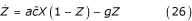In their equationis presented as "the proportion of bites by susceptible mosquitoes on infected people that produce a patent infection." This is interpreted to mean thatis the product of probability that a mosquito survives the incubation period, e-gn, and the transmission efficiency c. Substituting= ce-gn:= acXe-gn(1-Z) - gZ.     (27)

This equation would lead to a formula for R0 consistent with Eq. 22. However, solving for Z at equilibrium in Eq. 27 gives: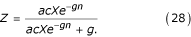This is not consistent with Eq. 8 (or formula 14.1a from ); thus, the statics are not consistent with the assumptions. The difference between Eq. 27 and Eq. 18 is the difference between e-gn(1 - Z) and (e-gn- Z). The former asserts that noninfectious mosquitoes, (1 - Z), become infectious at the rate acXe-gn, the latter that mosquitoes become infected at the rate acX, but only those who would survive the latent period, e-gn, ever become infectious. Eq. 18 is consistent with the other assumptions in the model. The two are equal if and only if the probability of surviving the incubation period, e-gn= 1.

## Appendix 2

There are two differences between the lines and symbols in Fig. 3. The first difference involves the definition and use of δ. The second issue involves the formula for the proportion of infectious mosquitoes.

Killeen, et al  define δ as the number of feeding cycles that would elapse before an infected mosquito becomes infectious. The mosquito would transmit on the subsequent bite. This would suggest that δ should always be the next largest integer (often the ceiling function). Killeen et al. always use the next smallest integer (often called the floor function). For example, if δ = 3.4, an infected mosquito would be infectious on the fourth subsequent bite, but Killeen et al. use the third bite. Which way is correct? Like the classic models, cyclical feeding models are also simple, deterministic approximations to much more complicated processes. Mosquitoes may feed at different rates, so the time to infection would be described by a distribution. Fig. 1 was generated using the average. One curve was produced using the smaller integer value of δ and a second curve using the larger integer. Next, the non-integer part of δ was used to weight the two curves. For example, for δ = 3.4, the upper curve was generated using δ = 3 and a lower curve using δ = 4, then 40% of the upper value was added to 60% of the lower value.

Killeen et al.  give a formula for the proportion of mosquitoes that are infectious, S i after surviving to a given feeding cycle, i. In their notation, k is the probability of infection per bite on an infectious human; thus k = cX. The proportion of blood meals on humans is Q. In their model, S i = kQ(i - δ), where δ is the number of feeding cycles that must elapse between becoming infected and becoming infectious. This formula is, at best, an approximation for short-lived mosquitoes when kQ is small; that is, it describes the proportion of infected mosquitoes as a linear function of the number of feeding cycles, and so encompasses the nonsensical possibility that more than 100% of mosquitoes can be infectious (see Fig. 3). Their formula for EIR (from Eq. 13 in Killeen, et al. ) is kLE/N h , where: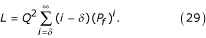where E is the daily emergence rate of adult mosquitoes and N h is human population density. The formula was rewritten, factoring (P f )δ and changing the index to j = i - δ, then taking the infinite sum: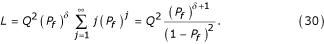Note that (P f )δ= P e . This formula does not match the one given by Saul, et al. . An exact formula for the proportion that are infectious after i feeding cycles is:

S i = 1 - (1 - kQ)i-δ    (31)

That is, to remain uninfected after i - δ feeding cycles, a mosquito must have failed to become infected by each one of the i - δ preceding bites. After δ feeding cycles have passed, the mosquito would be infectious. The exact formula is given by Saul, et al. (Table 2, ).

## References

1. Ross R: The prevention of malaria. 1911, London: John Murray

2. Macdonald G: The Epidemiology and Control of Malaria. 1957, London: Oxford University Press

3. McKenzie FE: Why model malaria?. Parasitol Today. 2000, 16: 511-6. 10.1016/S0169-4758(00)01789-0.

4. Anderson RM, May RM: Infectious Diseases of Humans. 1991, Oxford: Oxford University Press

5. Bailey NTJ: The Biomathematics of Malaria. 1982, Oxford University Press

6. Gillies MG, Wilkes TJ: A study of the age composition of populationas of Anopheles gambiae and An. funestus in North East Tanzania. Bull Ent Res. 1965, 56: 237-262.

7. Lines JD, Wilkes TJ, Lyimo EO: Malaria Infectiousness Measured by Age-Specific Sporozoite Rates in Anopheles gambiae Human in Tanzania. Parasitology. 1991, 102: 167-177.

8. Saul AJ, Graves PM, Kay BH: A cyclical feeding model for pathogen transmission and its application to determine vectorial capacity from vector infection rates. J Appl Ecol. 1990, 27: 123-133.

9. Killeen GF, McKenzie FE, Foy BD, Schieffelin C, Billingsley PF, Beier JC: A simplified model for predicting malaria entomologic inoculation rates based on entomologic and parasitologic parameters relevant to control. Am J Trop Med Hyg. 2000, 62: 535-544.

10. Fine PEM: Superinfection – A Problem in Formulating a Problem. Bureau of Hygiene and Tropical Diseases. 1975, 72: 475-488.

11. Aron JL, May RM: The population dynamics of malaria. In Population Dynamics and Infectious Disease. Edited by: Anderson RM. 1982, Chapman and Hall, 139-179.

12. Dietz K: Mathematical models for transmission and control of malaria. In Principles and Practice of Malariology. Edited by: Wernsdorfer W, McGregor I. 1988, Edinburgh: Churchill Livingston, Inc, 1091-1133.

13. Dye C, Hasibeder G: Population dynamics of mosquito-borne disease: effects of flies which bite some people more frequently than others. Trans Roy Soc Trop Med Hyg. 1986, 80: 69-77. 10.1016/0035-9203(86)90199-9.

14. Takken W, Knols BGJ: Odor-mediated behavior of Afrotropical malaria mosquitoes. Ann Rev Entomol. 1999, 44: 131-157. 10.1146/annurev.ento.44.1.131.

15. Smith T, Charlwood JD, Takken W, Tanner M, Spiegelhalter DJ: Mapping the densities of malaria vectors within a single village. Acta Trop. 1995, 59: 1-18. 10.1016/0001-706X(94)00082-C.

16. Hii JL, Smith T, Mai A, Mellor S, Lewis D, Alexander N, Alpers MP: Spatial and temporal variation in abundance of Anopheles (Diptera:Culicidae) in a malaria endemic area in Papua New Guinea. J Med Entomol. 1997, 34: 193-205.

17. Staedke SG, Nottingham EW, Cox J, Kamya MR, Rosenthal PJ, Dorsey G: Short report: proximity to mosquito breeding sites as a risk factor for clinical malaria episodes in an urban cohort of Ugandan children. Am J Trop Med Hyg. 2003, 69: 244-246.

18. van der Hoek W, Konradsen F, Amerasinghe PH, Perera D, Piyaratne MK, Amerasinghe FP: Towards a risk map of malaria for Sri Lanka: the importance of house location relative to vector breeding sites. Int J Epidemiol. 2003, 32: 280-285. 10.1093/ije/dyg055.

19. Davidson G: Estimation of the survival of Anopheline mosquitoes in nature. Nature. 1954, 174: 792-793.

20. Garrett-Jones C: Prognosis for interruption of malaria transmission through assessment of the mosquito's vectorial capacity. Nature. 1964, 204: 1173-1175.

21. Dye C, Williams BG: Non-Linearities in the Dynamics of Indirectly-Transmitted Infections (or, Does having a Vector make a Difference?). In Ecology of Infectious Diseases in Natural Populations. Edited by: Grenfell BT, Dobson AP. 1995, Cambridge, UK: Cambridge University Press, 260-279.

22. Dietz K, Molineaux L, Thomas A: A Malaria Model Tested in the African Savannah. B World Health Organ. 1974, 50: 347-357.

23. Box GEP: Robustness in the stratetgy of scientific model building. In Robustness in Statistics. Edited by: Launer RL, Wilkinson GN. 1979, New York: Academic Press

24. Dietz K: The estimation of the basic reproduction number for infectious diseases. Stat Methods Med Res. 1993, 2: 23-41.

25. Diekmann O, Heesterbeek JAP, Metz JAJ: On the definition and the computation of the basic reproduction ratio R0 in models for infectious diseases in heterogeneous populations. J Math Biol. 1990, 28: 365-382.

26. Snow RW, Gilles HM: The epidemiology of malaria. In Essential Malariology. Edited by: Warrell DA, Gilles HM. 2002, London: Arnold, 85-106. 4

27. Mutero CM, Birley MH: The effect of pre-gravid development on the estimation of mosquito survival rates. J Appl Ent. 1989, 107: 96-101.

28. Dye C: Epidemiological significance of vector-parasite interactions. Parasitology. 1990, 101: 409-415.

29. Dietz K: Models for vector-borne parasitic diseases. Lecture Notes in Biomathematics. 1980, 39: 264-277.

30. Killeen GF, Fillinger U, Knols BGJ: Advantages of larval control for African malaria vectors: Low mobility and behavioural responsiveness of immature mosquito stages allow high effective coverage. Malaria J. 2002, 1: 8-10.1186/1475-2875-1-8.

31. Molineaux L: Malaria and mortality: some epidemiological considerations. Ann Trop Med Parasit. 1997, 91: 811-825. 10.1080/00034989760572.

32. Cohen JE: Esitmating the effects of successful malaria control programmes on mortality. Popul Bull of the United Nations. 1988, 25: 6-26.

## Acknowledgments

We thank Wendy Prudhomme and Jonathan Dushoff for their comments.

## Author information

Authors

### Corresponding author

Correspondence to David L Smith.

### Authors' contributions

The authors contributed equally to this draft.

David L Smith and F Ellis McKenzie contributed equally to this work.

## Authors’ original submitted files for images

Below are the links to the authors’ original submitted files for images.

## Rights and permissions

Reprints and Permissions

Smith, D.L., Ellis McKenzie, F. Statics and dynamics of malaria infection in Anopheles mosquitoes. Malar J 3, 13 (2004). https://doi.org/10.1186/1475-2875-3-13

• Accepted:

• Published:

• DOI: https://doi.org/10.1186/1475-2875-3-13

### Keywords

• Malaria
• Malaria Transmission
• Stability Index
• Mosquito Population